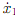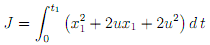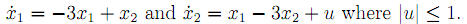## Find the optimal control, Data Structure & Algorithms

Assignment Help:

1. Use the Weierstrass condition, find the (Strongly) minimizing curve and the value of Jmin for the caseswhere x(1) = 0, x(2) = 3.

2. The system= x1 + 2u; where u = u(t) is not subject to any constraint, is to be controlled from x1(0) = 1 to x1(t1) = 2 where t1 is unspecified, in such as way thatis minimized. Find the optimal control.

Solve the problem of time-optimal control to the origin for the system#### Deletion of a node from an avl tree, For AVL trees the deletion algorithm i...

For AVL trees the deletion algorithm is a little more complicated as there are various extra steps involved in the deletion of node. If the node is not a leaf node, then it contain

#### Determine the disjoint of division method, Determine the Disjoint of divisi...

Determine the Disjoint of division method A polygon is disjoint from the viewport if the x- and y-extents of the polygon do not overlap the viewport anywhere. In this case; reg

#### Two sparce matrices multipilcation algorithm, Write an algorithm for multi...

Write an algorithm for multiplication of two sparse matrices using Linked Lists.

#### Circular linked list, In a circular linked list There is no beginning a...

In a circular linked list There is no beginning and no end.

#### Explain the assertions in ruby, Explain the Assertions in Ruby Ruby off...

Explain the Assertions in Ruby Ruby offers no support for assertions whatever. Moreover, because it's weakly typed, Ruby doesn't even enforce rudimentary type checking on opera

bfs and dfs

#### How many nodes in a tree have no ancestor, How many nodes in a tree have no...

How many nodes in a tree have no ancestors 1 node in atree have no ancestors.

#### Tree, tree is graph or not

tree is graph or not

#### Program segment for deletion of any element from the queue, Program segment...

Program segment for deletion of any element from the queue delete() { int delvalue = 0; if (front == NULL) printf("Queue Empty"); { delvalue = front->value;